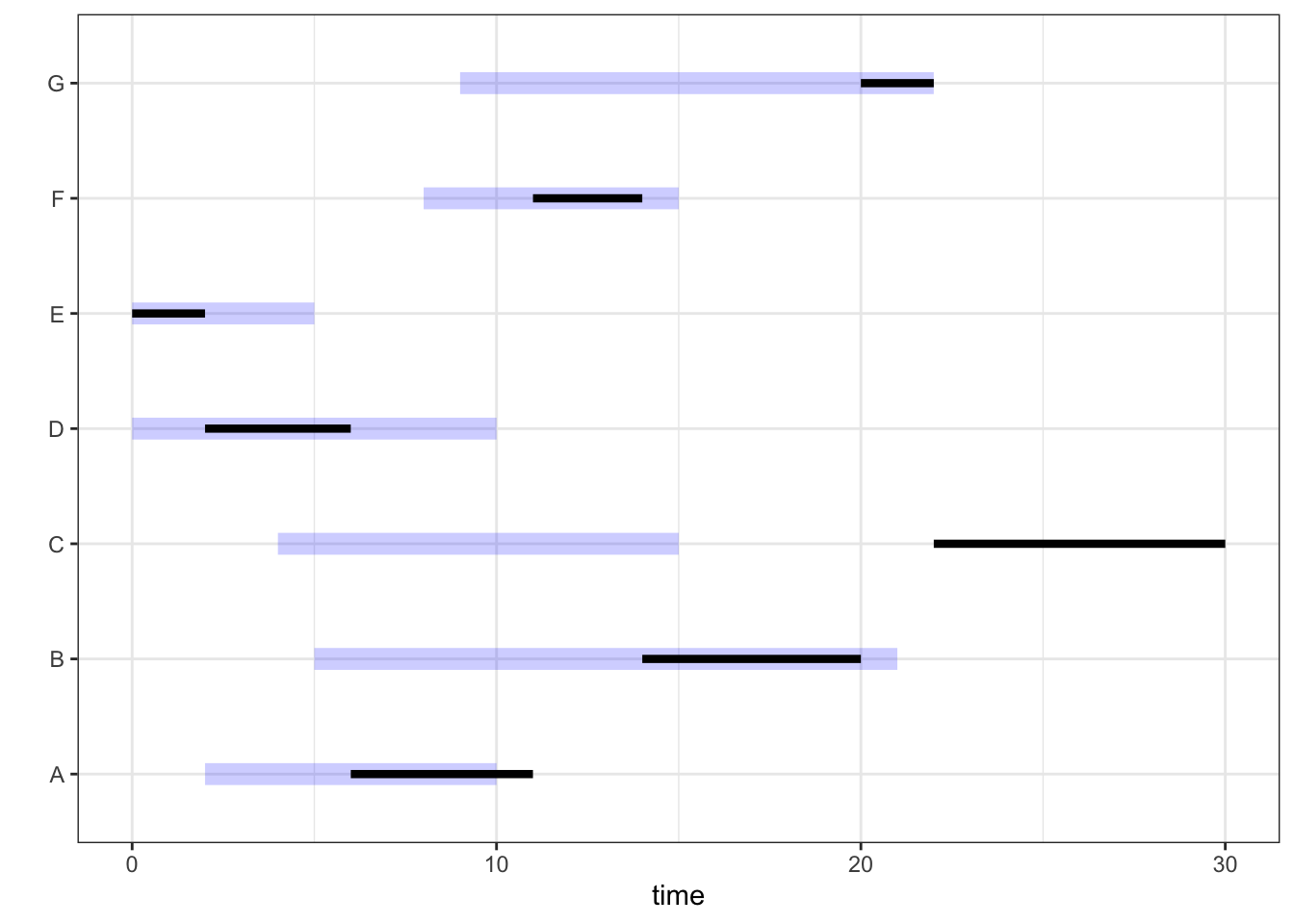#  Machine scheduling problem taken from Jeffrey Kantor's cookbook:
#  https://nbviewer.jupyter.org/github/jckantor/ND-Pyomo-Cookbook/blob/master/notebooks/04.01-Machine-Bottleneck.ipynb
#
#  https://jckantor.github.io/ND-Pyomo-Cookbook/
#

# set-up python version to use
reticulate::use_python("/opt/anaconda3/bin/python")
library(reticulate)

# import pyomo
pyo = import("pyomo.environ", convert = FALSE)
pygdp = import("pyomo.gdp", convert = FALSE)
bi = import_builtins()

# had signal handling issues when using solver. Turned
# off signal handling based on this discussion thread
# https://github.com/PyUtilib/pyutilib/issues/31
pyulib = import("pyutilib.subprocess.GlobalData")
pyulib$DEFINE_SIGNAL_HANDLERS_DEFAULT = FALSE # printing model to a file, reading it back into R to print in R markdown mdl_print = function(m) { printstr = " with open('tmp_model.txt', 'w') as f: r.mdl.pprint(ostream=f) f.close() " printstr = gsub("mdl", m, printstr) py_run_string(printstr) printstr_R = paste(readLines("tmp_model.txt"), collapse = "\n") return(printstr_R) } # get operators source("operators.R") # load other libraries library(dplyr) ## ## Attaching package: 'dplyr' ## The following objects are masked from 'package:stats': ## ## filter, lag ## The following objects are masked from 'package:base': ## ## intersect, setdiff, setequal, union library(ggplot2) # list of jobs to be scheduled on the single machine # with their release date, due date and duration of task jobs_df = tibble(job = c("A", "B", "C", "D", "E", "F", "G"), release = c(2, 5, 4, 0, 0, 8, 9), duration = c(5, 6, 8, 4, 2, 3, 2), due = c(10, 21, 15, 10, 5, 15, 22)) # data converted into list to be used in pyomo model release_L = list() duration_L = list() due_L = list() for (i in 1: nrow(jobs_df)) { release_L[[jobs_df$job[i]]] = jobs_df$release[i] duration_L[[jobs_df$job[i]]] = jobs_df$duration[i] due_L[[jobs_df$job[i]]] = jobs_df$due[i] } # max time tmax = sum(as.numeric(release_L)) + sum(as.numeric(duration_L)) # create model object M = pyo$ConcreteModel()

# Set of jobs
M$jobs = pyo$Set(initialize = jobs_df$job) # Set of job pairs (j, k) where j < k filt_rule = function(m, j, k) { return(j < k) } M$pairs = pyo$Set(initialize = M$jobs * M$jobs, filter = filt_rule) # Parameters: release, duration, due time M$release = pyo$Param(M$jobs, initialize = release_L)
M$duration = pyo$Param(M$jobs, initialize = duration_L) M$due = pyo$Param(M$jobs, initialize = due_L)

# decision variables for each job:start time
# other variables: time by which job is past due or early
M$start = pyo$Var(M$jobs, bounds = tuple(0, tmax)) M$pastdue = pyo$Var(M$jobs, bounds = tuple(0, tmax))
M$early = pyo$Var(M$jobs, bounds = tuple(0, tmax)) # objective: minimize total past due time across jobs obj_rule = function(m) { expr = 0 for (j in bi$list(m$jobs)) { expr = expr + m$pastdue[j]
}
return(expr)
}
M$obj = pyo$Objective(rule = obj_rule, sense = pyo$minimize) # constraint that enables calculation of past due time pastdue_calc_rule = function(m, j) { expr = m$start[j] + m$duration[j] + m$early[j] == m$due[j] + m$pastdue[j]
return(expr)
}
M$pastdue_calc_cons = pyo$Constraint(M$jobs, rule = pastdue_calc_rule) # constraint that start time of job >= release time of job release_rule = function(m, j) { expr = m$start[j] >= m$release[j] return(expr) } M$release_cons = pyo$Constraint(M$jobs, rule = release_rule)

#
# Disjunction that either (j precedes K) or (k precedes j)
#
ovlp_rule = function(m, j, k) {
expr1 = m$start[j] + m$duration[j] <= m$start[k] expr2 = m$start[k] + m$duration[k] <= m$start[j]
return(c(expr1, expr2))
}
M$ovlp_cons = pygdp$Disjunction(M$pairs, rule = ovlp_rule) # This is a mixed integer linear program (MILP) and # solved here using glpk pyo$TransformationFactory("gdp.hull")$apply_to(M) ## None res = pyo$SolverFactory('glpk')$solve(M) # the results are extracted into a tibble resL = list() for (i in bi$list(M$jobs)) { resL[[i]] = c(job = i, start = py_to_r(M$start[i]()), pastdue = py_to_r(M\$pastdue[i]()))
}
res_df = bind_rows(resL)
res_df = res_df %>% mutate(start = as.numeric(start), pastdue = as.numeric(pastdue))

res_df = inner_join(res_df, jobs_df, by = "job")
res_df = res_df %>% mutate(finish = start + duration)

# plot the results as a gantt chart
# light blue bars for a job start at release time and end at due time
#  solid line start at start time and end at start + duration time
#
p = ggplot(data = res_df)
p = p + geom_segment(aes(x = release, y = job, xend = due, yend = job), size = 4, alpha = 0.2, color = "blue")
p = p + geom_segment(aes(x = start, y = job, xend = finish, yend = job), size = 1.5)
p = p + labs(x = "time", y = "")
p = p + theme_bw()
p# In the optimal solution, C is past due by 15 time units and A is past due by 1 time unit

### Session Info

sessionInfo()
## R version 4.0.1 (2020-06-06)
## Platform: x86_64-apple-darwin17.0 (64-bit)
## Running under: macOS High Sierra 10.13.6
##
## Matrix products: default
## BLAS:   /Library/Frameworks/R.framework/Versions/4.0/Resources/lib/libRblas.dylib
## LAPACK: /Library/Frameworks/R.framework/Versions/4.0/Resources/lib/libRlapack.dylib
##
## locale:
##  en_US.UTF-8/en_US.UTF-8/en_US.UTF-8/C/en_US.UTF-8/en_US.UTF-8
##
## attached base packages:
##  stats     graphics  grDevices utils     datasets  methods   base
##
## other attached packages:
##  ggplot2_3.3.2   dplyr_1.0.0     reticulate_1.16
##
## loaded via a namespace (and not attached):
##   Rcpp_1.0.4.6     knitr_1.28       magrittr_1.5     munsell_0.5.0
##   tidyselect_1.1.0 colorspace_1.4-1 lattice_0.20-41  R6_2.4.1
##   rlang_0.4.6      stringr_1.4.0    highr_0.8        tools_4.0.1
##  grid_4.0.1       gtable_0.3.0     xfun_0.14        withr_2.2.0
##  htmltools_0.5.0  ellipsis_0.3.1   yaml_2.2.1       digest_0.6.25
##  tibble_3.0.1     lifecycle_0.2.0  crayon_1.3.4     Matrix_1.2-18
##  farver_2.0.3     purrr_0.3.4      vctrs_0.3.1      glue_1.4.1
##  evaluate_0.14    rmarkdown_2.3    labeling_0.3     stringi_1.4.6
##  compiler_4.0.1   pillar_1.4.4     scales_1.1.1     generics_0.0.2
##  jsonlite_1.6.1   pkgconfig_2.0.3
# pyomo version used is 5.7